# NABARD Manager Reasoning Mock Test 9

## 20 Questions MCQ Test NABARD Manager - Mock Tests & Previous Year Papers | NABARD Manager Reasoning Mock Test 9

Description
Attempt NABARD Manager Reasoning Mock Test 9 | 20 questions in 12 minutes | Mock test for Banking Exams preparation | Free important questions MCQ to study NABARD Manager - Mock Tests & Previous Year Papers for Banking Exams Exam | Download free PDF with solutions
QUESTION: 1

### A, B, C, D and E are sitting on a bench. A is sitting next to B, C is sitting next to D, D is not sitting with E who is on the left end of the bench. C is on the second position from the right. A is to the right of B and E. A and C are sitting together. In which position A is sitting ?

Solution:
Therefore, A is sitting in between B and C.
QUESTION: 2

### Four girls are sitting on a bench to be photographed. Shikha is to the left of Reena Manju is to the right of Reena. Rita is between Reena and Manju. Who would be second from the left in the photograph?

Solution:

Shikha is to the left of Reena and Manju is to her right.
Reeta is between Reena and Manju
So, the order is
Shikha - Reena - Rita - Manju
In the photograph, Rita will be second from left

QUESTION: 3

### The government of Zunimagua has refused to schedule free elections, release political prisoners, or restore freedom of speech; therefore, no more financial aid from the United States should be provided to Zunimagua. Which of the following is an assumption made in the argument above?

Solution:
QUESTION: 4

Journalism professor: Since the number of reportable facts confronting any journalist is extraordinarily large, every journalist must employ a principle of selection. However, any such principle of selection is bound to be subjective, for it involves value judgments. Therefore, the view that there is any degree of objectivity in journalistic reporting is a myth. This means that, for example, no newspaper article is more objective than any other.
The journalism professor’s argument is flawed because it

Solution:
QUESTION: 5

Patel: It is often thought that direct experience, unlike language and culture, can always serve as common ground for communication among human beings. But people from different cultures inhabit different sensory worlds. Because selective screening of sensory data admits some perceptions while filtering out others, one person’s experience, perceived through one set of culturally patterned sensory screens, is quite different from what anyone from another culture would experience when encountering the same sensory data.
Which one of the following is an assumption required by Patel’s argument?

Solution:
QUESTION: 6
Below are the statements followed by two conclusions numbered I and II. You have to consider the statements and the following conclusions and decide which of the conclusion(s) follows the statement(s).
Statements :
a. Some cars are wheels.
b. All wheels are branches.
c. Some branches are tins.
Conclusions :
I.Some cars are branches.
II. Some tins are branches.
Solution:
Conclusions:
(I) True
(II) True
Hence, both the statements are true
QUESTION: 7

In the following questions, the symbols αα,ββ,δδ,γγ and ηη are used with the following .
'P αα Q' means 'P greater than Q'
'P ββ Q' means 'P is either greater than or equal to Q'.
P δδ Q means P is equal to Q.
P γγ Q means P is smaller then Q.
P ηη Q means P is either smaller than or equal to Q.
Now in each of the following questions assuming the given statements to be true, find out which of the two conclusions I and II given below them is/are definitely true?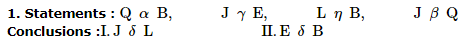Solution: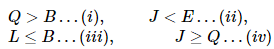By an intelligent observation we find there is no sign of δδ in any equation given in the statement. Hence, we can conclude within a second that both I and II are not true.

QUESTION: 8

In the following questions, the symbols αα,ββ,δδ,γγ and ηη are used with the following .
'P αα Q' means 'P greater than Q'
'P ββ Q' means 'P is either greater than or equal to Q'.
P δδ Q means P is equal to Q.
P γγ Q means P is smaller then Q.
P ηη Q means P is either smaller than or equal to Q.
Now in each of the following questions assuming the given statements to be true, find out which of the two conclusions I and II given below them is/are definitely true?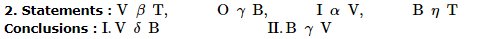Solution: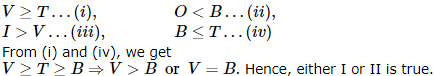QUESTION: 9

In the following questions, the symbols αα,ββ,δδ,γγ and ηη are used with the following .
'P αα Q' means 'P greater than Q'
'P ββ Q' means 'P is either greater than or equal to Q'.
P δδ Q means P is equal to Q.
P γγ Q means P is smaller then Q.
P ηη Q means P is either smaller than or equal to Q.
Now in each of the following questions assuming the given statements to be true, find out which of the two conclusions I and II given below them is/are definitely true?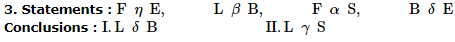Solution:

Again, by an intuitie look we come to know L is not equal to nay element in the given equations in the statements. Hence, I is not true. Again, as you have in our Magiical Book Series on Analytical Reasoning by MK Pandey, Tip 8 say 'If a term was not less than (or, less than or equal to') any other term in the given statements, then it can't be less than (or, "less than or equal to") any term in a conclusion. (Such a conclusion is definitely false) Thus II is not true.

QUESTION: 10

Ten members I, J, K, L, M, N, O, P, Q and R are split into two teams X and Y of 5 members each. Members in the two teams are made to sit in two rows facing each other in such a way that one member of team X is sitting exactly opposite of a member in team Y. Members of team X are facing North. L is third to the right of I and sits exactly opposite of O. J sits to the immediate right of O and is facing South. P is third to the right of J and is exactly opposite of N. K sits between I and M and is opposite of Q.

Q. Who is second to the left of K?

Solution: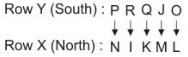QUESTION: 11

Ten members I, J, K, L, M, N, O, P, Q and R are split into two teams X and Y of 5 members each. Members in the two teams are made to sit in two rows facing each other in such a way that one member of team X is sitting exactly opposite of a member in team Y. Members of team X are facing North. L is third to the right of I and sits exactly opposite of O. J sits to the immediate right of O and is facing South. P is third to the right of J and is exactly opposite of N. K sits between I and M and is opposite of Q.

Q. Which of the following group of persons sit in the same row?

Solution:QUESTION: 12

Ten members I, J, K, L, M, N, O, P, Q and R are split into two teams X and Y of 5 members each. Members in the two teams are made to sit in two rows facing each other in such a way that one member of team X is sitting exactly opposite of a member in team Y. Members of team X are facing North. L is third to the right of I and sits exactly opposite of O. J sits to the immediate right of O and is facing South. P is third to the right of J and is exactly opposite of N. K sits between I and M and is opposite of Q.

Q. Who is the immediate right of J?

Solution:QUESTION: 13

Each question below consists of two statements numbered I and II . You have to decide whether the data provided in the statements are sufficient to answer the questions.
Give answer (1) if the statement I alone is sufficient to answer the question, but the statement II alone is not sufficient.
Give answer (2) if the statement II alone is sufficient to answer the question, but the statement I alone is not sufficient.
Give answer (3) if both statements I and II together are needed to answer the question.
Give answer (4) if you cannot get the answer from the statement I and II together, but need even more data.

Q. What is the area of a rectangular field whose length is 40 meter?
I. A fence around the entire boundary of the field is 140 meter long.
II. The field is more than 20 meters wide.

Solution:

Area = ?
l = 40
⇒ 2(l + b) = 140
⇒ l + b = 70
⇒ b = 30 units
Area = lb
= (30) (40)
= 1200 units
Hence only statement 1 is sufficient to answer the question

QUESTION: 14

Each question below consists of two statements numbered I and II . You have to decide whether the data provided in the statements are sufficient to answer the questions.
Give answer (1) if the statement I alone is sufficient to answer the question, but the statement II alone is not sufficient.
Give answer (2) if the statement II alone is sufficient to answer the question, but the statement I alone is not sufficient.
Give answer (3) if both statements I and II together are needed to answer the question.
Give answer (4) if you cannot get the answer from the statement I and II together, but need even more data.

Q. Is a given rectangular block, a cube?
I. At least 2 faces of the rectangular block are squares.
II. The volume of the block is 64.

Solution:

We cannot tell whether the given block is a cube, as the statements provided are not sufficient

QUESTION: 15

Each question below consists of two statements numbered I and II . You have to decide whether the data provided in the statements are sufficient to answer the questions.
Give answer (1) if the statement I alone is sufficient to answer the question, but the statement II alone is not sufficient.
Give answer (2) if the statement II alone is sufficient to answer the question, but the statement I alone is not sufficient.
Give answer (3) if both statements I and II together are needed to answer the question.
Give answer (4) if you cannot get the answer from the statement I and II together, but need even more data.

Q. Is x3 equal to 125?
I. x>4
II. x<6

Solution:

Using both statements
4 < x < 6
⇒ x = 5
Hence, both statements are needed to answer the question

QUESTION: 16

A word arrangement machine, when given an input line of words, rearranges them following a particular rule in each step. The following is the illustration of the input and the steps of arrangement.
Input: man's mood varies with time and environment
Step I: varies with man's mood environment and time
Step II: and time environment mood man's varies with
Step III: environment time and varies with mood man's
Step IV: and varies environment time man's mood with
And so on for subsequent steps. You have to find out the logic and answer the questions given below:

Q. If Step V reads "bees are sucking juice from colourful flowers", what would Step III read?

Solution:
QUESTION: 17

A word arrangement machine, when given an input line of words, rearranges them following a particular rule in each step. The following is the illustration of the input and the steps of arrangement.
Input: man's mood varies with time and environment
Step I: varies with man's mood environment and time
Step II: and time environment mood man's varies with
Step III: environment time and varies with mood man's
Step IV: and varies environment time man's mood with
And so on for subsequent steps. You have to find out the logic and answer the questions given below:

Q. If Step III reads "old streets of Calcutta attract me lots", what would be the arrangement for Step VII?

Solution:
QUESTION: 18

A word arrangement machine, when given an input line of words, rearranges them following a particular rule in each step. The following is the illustration of the input and the steps of arrangement.
Input: man's mood varies with time and environment
Step I: varies with man's mood environment and time
Step II: and time environment mood man's varies with
Step III: environment time and varies with mood man's
Step IV: and varies environment time man's mood with
And so on for subsequent steps. You have to find out the logic and answer the questions given below:

Q. If Step IV reads "everyone were aware about their intimate friendship", what will be the middle three words of Step II?

Solution:
QUESTION: 19

Below are the statements followed by two conclusions numbered I and II. You have to consider the statements and the following conclusions and decide which of the conclusion(s) follows the statement(s).

Statements:
a. All cards are envelopes.
b. All cards are aerogrammes.
Conclusions:
I. All aerogrames are envelopes.
II. Some envelopes are aerogrames.

Solution: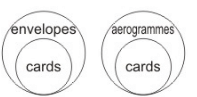Conclusions:
(I) False
(II) False
Beither conclusion follows

QUESTION: 20

Below are the statements followed by two conclusions numbered I and II. You have to consider the statements and the following conclusions and decide which of the conclusion(s) follows the statement(s).

Statements:
a. Some balls are bats.
b. Some balls are sticks.
Conclusions:
I. Every ball is either bat or stick.
II. Some balls are neither bat nor stick.

Solution: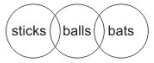Conclusions:
(I) False
(II) False
Neither conclusion followsUse Code STAYHOME200 and get INR 200 additional OFF Use Coupon Code